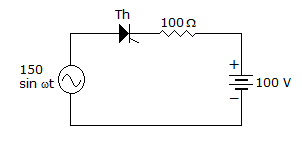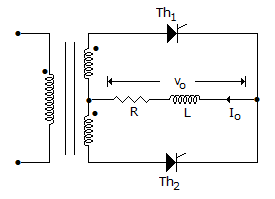# Electronics and Communication Engineering - Power Electronics

Exercise : Power Electronics - Section 8
1.
In a 3 phase bridge inverter, the gating signals for the three phases have a phase difference of
120°
60°
240°
any of the above
Explanation:
No answer description is available. Let's discuss.

2.

Assertion (A): The circuit of figure thyristor will conduct for 180° during positive half cycle if it is continuously fired.Reason (R): A thyristor can conduct only when it is forward biased.

Both A and R are correct and R is correct explanation of A
Both A and R correct but R is not correct explanation of A
A is correct but R is wrong
A is wrong but R is correct
Explanation:
No answer description is available. Let's discuss.

3.
The total number of leads in SCR, DIAC and TRIAC respectively are
3, 2, 3
2, 3, 3
3, 3, 2
3, 2, 4
Explanation:
No answer description is available. Let's discuss.

4.
A single phase semiconverter is feeding a highly inductive load and has a freewheeling diode across the load. The waveshape of input current is
sinusoidal
rectangular
sinusoidal or rectangular
triangular